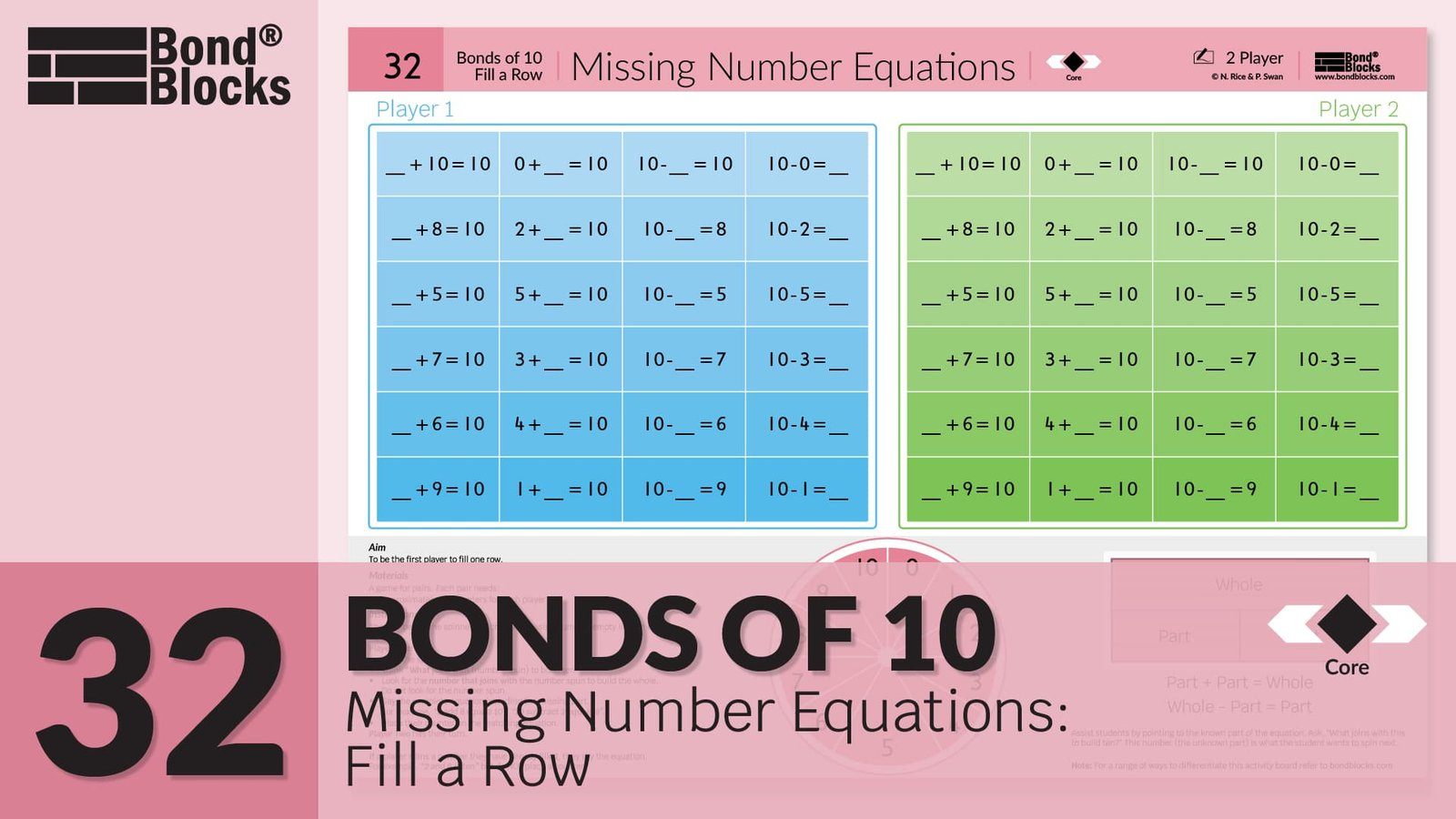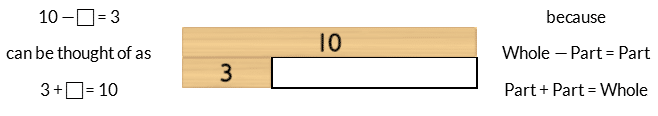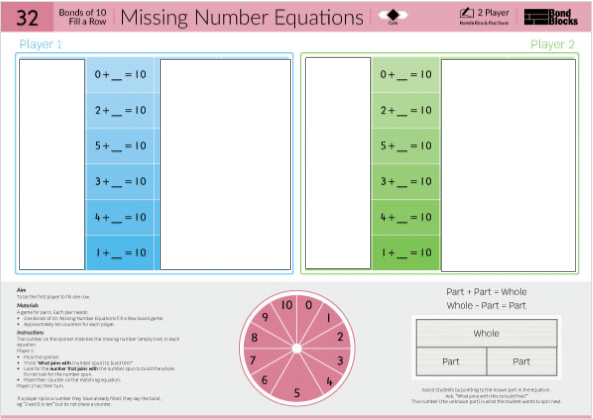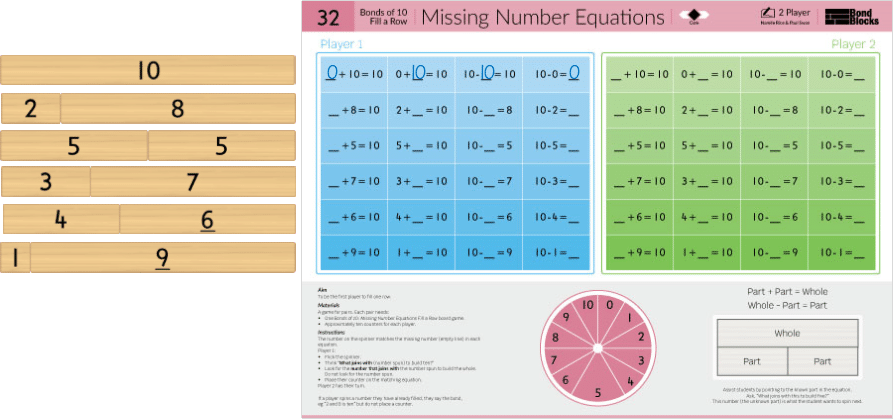## 32) Missing Number Equations

Bonds of 10: Fill a Row### Mathematics

Develop fluency using two-part bonds of 10 in related addition or subtraction equation where the unknown is a missing part.

### Language

• subtraction as ‘subtract’. Read aloud as “(Whole) subtract (part) equals (part)”.
• equals
• part-part-whole
• Subtraction solved using adding on. For example, 10 – ? = 3 can be thought of as “What part joins (adds on) to 3 to make the whole of 10?”
• The activity board uses the word “missing”. The word “unknown” is introduced when the Missing Number Sorting Cards are used.## Differentiation

### A little easier

##### One column play

Play using the first column on each player’s game board. The player who fills the most missing numbers in 3 minutes wins. This is helpful for students who need to focus on repeating the same type of question to develop fluency.Repeat using different columns. Each column has the missing number in a different position.##### Build a wall
• Build a two-part bond wall of 10. Use one of each block from 1 to 10 and one additional 5 block. Place this next to each related row on the activity board.
• Use the blocks to solve each missing number in the first row. Write each missing number on the write and wipe sleeve with a dry erase marker.
• Repeat for each row.
• Discuss patterns. For example, each row uses the same two-part bond of 10.##### Card sort

Click to download the Bonds of 10 Missing Number Equations Card Sort. Each card is one of the equations from the Bonds of 10 Missing Number Equations game board. Instruct students to:

Use the first two columns only. These cards contain a missing part.

• Cut out each card.
• Sort the cards into two groups (one of addition, the other subtraction) using the diagram heading cards.• Build a two-part bond wall of 10. Use one of each block from 1 to 10 and one additional 5 block.
• Start with the addition cards. Select one row from the Bond Block two-part Bonds of 10 wall. Search the addition cards for related equations. Fill in the missing number and place these cards next to the related Bond Blocks. Repeat for each row until all the missing number addition cards have been filled in and placed next to the related row of Bond Blocks.
• Repeat for the subtraction cards with a missing part.
• Discuss strategies for calculating the unknown part, noting its different position in addition and subtraction equations.### A little harder

##### Card sort

Click to download the Bonds of 10 Missing Number Equations Card Sort.

Use the all three columns of cards. The cards in the third column contain a missing whole in a subtraction equation.

Instruct students to:

• Cut out each card.
• Mix the cards.
• Sort the cards into three groups using the diagram heading cards:
• subtraction: one part unknown
• subtraction: whole unknown

Ensure the order of cards within each column is in a random order to make this activity a little harder. Instruct students to:

• Build a two-part bond wall of 10. Use one of each block from 1 to 10 and one additional 5 block.
• Start with the addition cards. Select one row from the Bond Block two-part Bonds of 10 wall. Search the addition cards for related equations. Fill in the missing number and place these cards next to the related Bond Blocks. Repeat for each row until all the missing number addition cards have been filled in and placed next to the related row of Bond Blocks.
• Repeat for the subtraction cards with a missing part.
• Repeat for the subtraction cards with a missing whole.
• Discuss strategies for calculating the unknown, including the importance of first identifying if it is a missing part or whole.##### Missing number equations tic-tac-toe

Play Bonds of 10: Missing Number Equations Tic-Tac-Toe a little harder. This game board is harder in two ways when compared to the core game board:

• The missing number equations aren’t grouped in related bonds but instead are placed randomly on the board.
• It includes subtraction equations where the missing number is in the whole position.• Support students who are having difficulty by clicking to download the Bonds of 10 Missing Number Equations Card Sort. Cut the cards and sort them using the three header cards. Use the cards grouped under the Subtraction Whole Unknown header card to identify subtraction equations on the game board where the whole is the unknown. Place the game board in a write and wipe sleeve and circle these equations. Then play.### Progression

In the next activity students apply the skills of representing of Bonds of 10 with Bond Blocks and as addition and subtraction equations to create related number stories using words and pictures. Go to

##### Activity 33

Bonds of 10: Representing Addition, Thinkboard

Bonds of 10: Representing Subtraction, Thinkboard